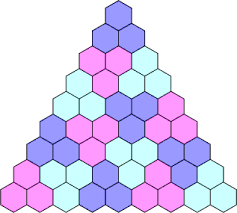# Three members AP

There are arithmetic sequence x + 3, 2x + 3, 5x - 3. If the common difference is 3, find x.

x =  3

### Step-by-step explanation:Did you find an error or inaccuracy? Feel free to write us. Thank you!

Tips for related online calculators
Do you have a linear equation or system of equations and looking for its solution? Or do you have a quadratic equation?